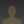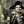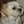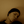0%
0%

# JS "Art"

Javascript Generated "Art"

Similar projects worth following
2.7k views
Just bunch of dots connected by Delaunay algorithm to triangles. From wikipedia:

In mathematics and computational geometry, a Delaunay triangulation for a set P of points in a plane is a triangulation DT(P) such that no point in P is inside the circumcircle of any triangle in DT(P). Delaunay triangulations maximize the minimum angle of all the angles of the triangles in the triangulation; they tend to avoid skinny triangles. The triangulation is named after Boris Delaunay for his work on this topic from 1934.

js triangles for all !

### wallpapers.zip

all in one place

Zip Archive - 28.53 MB - 03/15/2016 at 18:01

### 07-nexus-6.png

image/png - 2.74 MB - 12/18/2015 at 23:52

### 03-nexus-6.png

image/png - 656.11 kB - 12/18/2015 at 23:52

### 01-nexus-6.png

image/png - 1.29 MB - 12/18/2015 at 23:52

### 02-iphone-5.png

image/png - 247.61 kB - 12/18/2015 at 23:52

• ### functions

Ivan Lazarevic06/08/2015 at 19:22 0 comments

```/**
* Create random dots
* @param  {[type]} no number of dots
* @return {[type]}    [description]
*/
function random(no) {
var dots = [
[0, 0],
[0, height],
[width, 0],
[width, height]
]; // corner dots

for (var i = 0; i < no / 20; i++) {
dots.push([0, Math.random() * height]);
dots.push([Math.random() * width, height]);
dots.push([width, Math.random() * height]);
dots.push([Math.random() * width, 0]);

}

for (var i = 0; i < no - no / 20; i++) {
dots.push([Math.random() * width, Math.random() * height]);
}

return dots;

}```
```/**
* Put dots in spiral
* @param  {[type]} no     [description]
* @param  {[type]} factor [description]
* @return {[type]}        [description]
*/
function spiral(no, factor) {

var dots = [];

no = no || 1000;
factor = factor || 1;

for (i = 0; i < no; i++) {
angle = factor * i;
x = (20 + angle) * Math.cos(angle);
y = (40 + angle) * Math.sin(angle);
dots.push([x + width / 2, y + height / 2]);
}

return dots;

};```

• ### Triangles

Ivan Lazarevic06/04/2015 at 18:28 2 comments
• 1
Step 1

First, we need function to create dots which will be used for creating triangles. Easiest way is to create some dots by random. We gonna need width and height of canvas and number of dots and we can create array of random dots that will fit on canvas.

```var width = 800;
var height = 600;
var no = 10;
var dots = [];

for (var i = 0; i < no; i++) {
dots.push([Math.random() * width, Math.random() * height]);
}```
• 2
Step 2

After creating dots we’ll use Delaunay algorithm to connect dots to triangles. From wikipedia:

In mathematics and computational geometry, a Delaunay triangulation for a set P of points in a plane is a triangulation DT(P) such that no point in P is inside the circumcircle of any triangle in DT(P). Delaunay triangulations maximize the minimum angle of all the angles of the triangles in the triangulation; they tend to avoid skinny triangles. The triangulation is named after Boris Delaunay for his work on this topic from 1934.

There is a nice JavaScript implementation for that done by ironwallaby. That module and algorithm are core of what we gonna do, everything else is just a makeup.

`var triangles = Delaunay.triangulate(dots);`
• 3
Step 3

We’ll use canvas to draw triangles, so you’ll need canvas element in your html file, something like this:

`<canvas id="triglici"></canvas>`

Share

## Discussions

Yann Guidon / YGDES wrote 05/01/2017 at 20:44 point

I should definitely find the time to play with this code.

One day :-P

Are you sure? yes | no

Richard Hogben wrote 06/29/2015 at 22:57 point

Tried something :PAre you sure? yes | no

Ivan Lazarevic wrote 07/29/2015 at 19:09 point

looks nice.

Are you sure? yes | no

Ivan Lazarevic wrote 06/08/2015 at 19:20 point

most important part is this lib https://github.com/ironwallaby/delaunay everything else is just makeup : )

api page bg is just a static image for now.

Are you sure? yes | no

Alex Rich wrote 06/06/2015 at 00:26 point

so are you going to show us how you do it?  the one you made for the api page background is cool, is it an image or dynamically generated every time?

Are you sure? yes | no

## Similar Projects

Project Owner Contributor

### Polargraph type hot wire foam cutterAdam

Project Owner Contributor

### Understanding and Designing 'Neural' NetworksTegwyn☠Twmffat

Project Owner Contributor

### A Simple DXF Output Libraryagp.cooper

Project Owner Contributor

### SNES Jurassic Park computer terminal fractalstehaxor69

# Does this project spark your interest?

Become a member to follow this project and never miss any updates